4901.0 - Children's Participation in Cultural and Leisure Activities, Australia, Apr 2012
Latest ISSUE Released at 11:30 AM (CANBERRA TIME) 31/10/2012
 Page tools: .mffeedback,#pjs { display :none; } Enable Javascript to Print PagesPrint AllEmail NotificationRSSSearch this Product Explanatory Notes Glossary Abbreviations Quality Declaration Data quality (Technical Note) Data Source TECHNICAL NOTE DATA QUALITY RELIABILITY OF THE ESTIMATES 1 Since the estimates in this publication are based on information obtained from a sample, they are subject to sampling variability. That is, they may differ from those estimates that would have been produced if all dwellings had been included in the survey. One measure of the likely difference is given by the standard error (SE), which indicates the extent to which an estimate might have varied by chance because only a sample of dwellings (or households) was included. There are about two chances in three (67%) that a sample estimate will differ by less than one SE from the number that would have been obtained if all dwellings had been included, and about 19 chances in 20 (95%) that the difference will be less than two SEs. 2 Another measure of the likely difference is the relative standard error (RSE), which is obtained by expressing the SE as a percentage of the estimate.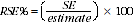3 RSEs for CPCLA have been calculated using the Jackknife method of variance estimation. This involves the calculation of 30 'replicate' estimates based on 30 different sub-samples of the obtained sample. The variability of estimates obtained from these sub-samples is used to estimate the sample variability surrounding the estimate. 4 RSEs of all the estimates in this publication are included in the Datacubes released as part of the publication and available from the Downloads tab of the publication. 5 Table 1 contains estimates collected from previous Children's Participation in Cultural and Leisure Activities surveys. The spreadsheets associated with this release contain RSEs for these estimates. The RSEs for 2006 were calculated using a different statistical SE model, which is available from the 2006 Children's Participation in Cultural and Leisure Activities, Australia (cat no. 4901.0) and on the ABS website . From the 2009 survey onwards, the RSEs were directly calculated for each separate estimate. While the direct method is more accurate, the difference between the two methods is usually not significant for most estimates. 6 Only estimates (numbers and proportions) with RSEs less than 25% are considered sufficiently reliable for most purposes. Estimates with RSEs between 25% to 50% have been included with a cell comment to indicate they are subject to high sample variability and should be used with caution. Estimates with RSEs greater than 50% are included with a cell comment to indicate that they are considered too unreliable for general use. CALCULATION OF STANDARD ERROR 7 Standard errors can be calculated using the estimates (counts or proportions) and their corresponding RSEs. For example Table 5 shows the estimated number of children who participated in playing a musical instrument was 490,200. The RSE Table corresponding to the estimates in Table 5 (included in the Datacubes in the Downloads tab) shows the RSE for this estimate is 3.1%. The SE is calculated by: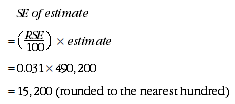8 Therefore, there are about two chances in three that the value that would have been produced if all children had been included in the survey will fall within the range 475,000 to 505,400 and about 19 chances in 20 that the value will fall within the range 459,800 to 520,600. This example is illustrated in the diagram below: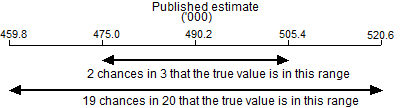9 In general, the size of the SE increases as the size of the estimate increases. Conversely, the RSE decreases as the size of the estimate increases. Very small estimates are subject to such high RSEs that their value for most practical purposes is unreliable and should only be used to aggregate with other estimates to provide derived estimates with RSEs of less than 25%. CALCULATION OF STANDARD ERROR FOR MEANS AND MEDIANS 10 This publication contains means and medians. Both are measures for locating the centre of a set of values, but each measure has its own method of calculation. The mean is the arithmetic average, whereas the median is the middle value of a set of values when the values are sorted in size order. 11 Table 5 shows that the estimated number of children who played a musical instrument was 490,200 with the median number of hours those children played a musical instrument being 3 hours in the last 2 weeks of school. 12 Standard errors can be calculated using the estimates (means or medians) and their corresponding RSEs. The RSE table corresponding to the estimates in Table 5 (see Datacubes in the Downloads tab) shows the RSE for the estimated median number of hours children played a musical instrument in the last 2 weeks of school is 8.5%. The SE is calculated by: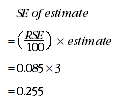13 Therefore, there are about two chances in three that the value that would have been produced if all children had been included in the survey will fall within the range 2.7 and 3.3 and about 19 chances in 20 that the value will fall within the range 2.5 to 3.5. This example is illustrated in the diagram below: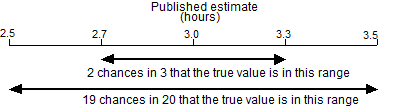DIFFERENCES 14 Published estimates may also be used to calculate the difference between two survey estimates (of numbers or proportions). Such an estimate is also subject to sampling error. The sampling error of the difference between two estimates depends on their SEs and the relationship (correlation) between them. An approximate SE of the difference between two estimates (x-y) may be calculated by the formula: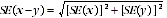15 While this formula will only be exact for differences between separate and uncorrelated (unrelated) characteristics of sub-populations, it is expected to provide a good approximation for all differences likely to be of interest in this publication. SIGNIFICANCE TESTING 16 A statistical significance test for any comparisons between estimates can be performed to determine whether it is likely that there is a true difference between the corresponding population characteristics. The standard error of the difference between two corresponding estimates (x and y) can be calculated using the formula above. This standard error is then used to calculate the following test statistic: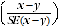17 If the value of this test statistic is greater than 1.96 then there is evidence, with a 95% level of confidence, of a statistically significant difference in the two between the populations with respect to that characteristic. Otherwise, it cannot be stated with confidence that there is a real difference between the populations with respect to that characteristic. Document Selection These documents will be presented in a new window.